# Chapter 9 - Boolean operators, and more about the return statement¶

This section introduces the boolean operators represented by the Python keywords and, or, and not. Boolean operators enable us to simplify the logic of conditional statements by combining boolean expressions.

## 1.1 The boolean operators¶

Let’s start by looking at an example that we used in a previous chapter: Mousetraps are sold online for 2.00 each plus .50 for shipping, with free shipping for orders of 30 or more. The logic that we used to calculate the shipping could be expressed like this (where num refers to the number ordered):

if num >= 30:
shipping_cost = 0
else:
shipping_cost = num * .50


Now suppose that shipping is also free for customers in zip code 50011. So before computing the shipping cost, we have to check the zip code. Assume that zip_code is a variable representing the zip code.

if num >= 30:
shipping_cost = 0
else:
if zip_code == 50010:
shipping_cost = 0;
else:
shipping_cost = num * .50


This makes sense, but doesn’t it seem a bit strange that the line shipping_cost = 0appears in two places?

After all, there are only two possibilities, either the shipping cost is zero, or else it’s num times 50 cents. It seems like what we really want to say is something like this: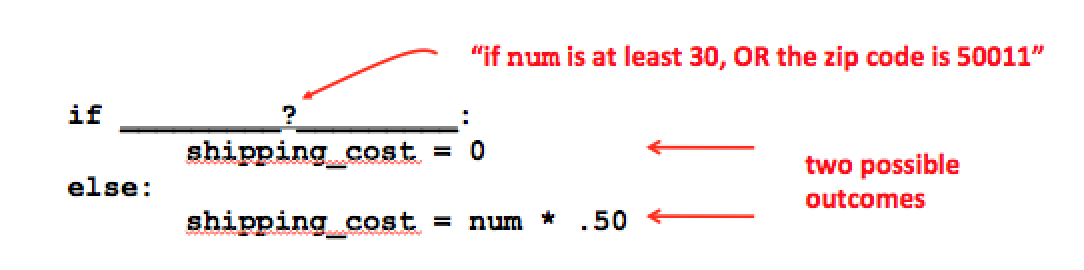So what goes in the blank? Logically we want to say “if num is at least 30 or the zip code is 50011, then the shipping is free”. Well, that’s the purpose of the or operator in Python. We can just write:

if num >= 30 or zip_code == 50010:
shipping_cost = 0
else:
shipping_cost = num * .50


To define exactly what the or operator does, let’s first summarize the possibilities and list the outcomes we intend. Each of the expressions can be either true or false, so there are four possibilities.

If num is at least 30 and the zip code is 50011, then shipping is free.

If num is at least 30 and the zip code isn’t 50011, then shipping is free.

If num is less than 30 but the zip code is 50011, then shipping is free.

Finally if num is less than 30 and the zip code isnt 50011, then shipping is not free.This table tells us under what conditions this new expression should be true.

In fact, we can use the same table to define the or operator for any two boolean expressions A and B.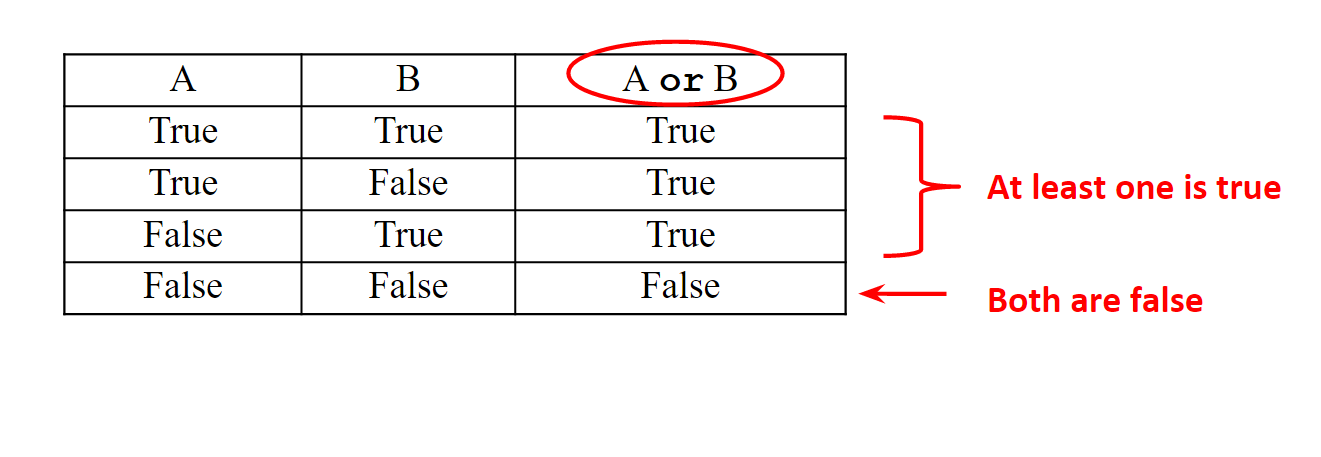The table tells us that the new expression A or B is true if at least one or A or B is true. This is called the truth table for the or operator.

Let’s try another example. Suppose we have a function print_grade that prints the grade for a given score, but we first have to check that the score is between 0 and 100.

We could write something like this:

if score >= 0:
if score<100
else:
print("out of range")
else:
print("out of range")


But again, it seems odd to have the same print statement appearing in two places. There are really only two possible outcomes, so we should be able to write something like this: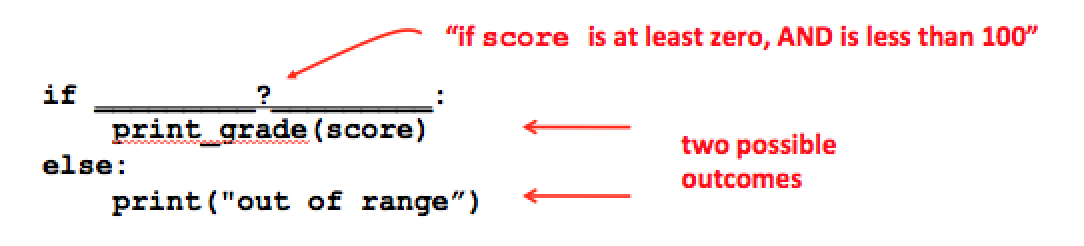Now, what goes in the blank? We are trying to say “If score is above zero and less than 100, then print the grade.” That’s the purpose of the and keyword in Python:

if score >= 0 and score <= 100:
else:
print("out of range")


The new expression is true just when both of the original expressions are true. The and operator is defined with the following truth table.The way that the and keyword is used corresponds to the way we use the word “and” in English. If someone says “My date was rich and handsome,” under what conditions is this a true statement? The guy might be rich, the guy might be handsome, but the statement “My date was rich and handsome” is only true when he’s both. Poor and handsome, or rich and ugly, just won’t cut it.

The way that the or keyword works is similar to the word “or” in English, but only when it is used in the “inclusive” sense. If you ask someone, “Are you crazy or just plain stupid?” and he says yes, it means he might be crazy, he might be stupid, but he might be both, and his answer “yes” would be true in all three cases. That’s the inclusive “or”. On the other hand, when the waiter asks you, “Would you like the soup or the salad?” he usually means you can choose a soup, OR a salad, but not both. That’s the exclusive “or”. The or keyword works like the inclusive “or” in English.

There is one more boolean operator, called not. Unlike the and and or operators, the not operator doesn’t combine two expressions. It applies to just one expression. Just like you can take a number put a minus sign in front of it, and get the negative of the number, you put not in front of any boolean expression to get the opposite value.

For example, the expression

not(x > 0)

has the same meaning as

x <= 0

Likewise, the expression

not(x == 100)

means the same thing as

x != 100

not(score >= 0 and score <= 100)

You can read this as, “it is not the case that score is between 0 and 100”, which is the same as saying the score is less than 0 or is greater than 100:

score < 0 or score > 100

In all these examples, it was easy to rewrite the expression so that it doesn’t use the not operator. In some cases it is not so simple. Here is an example in which the not operator is extremely useful. We previously wrote a function is_divisible(a, b) that returns True when a is divisible by b and False otherwise. What if, given two values x and y, we want to say, “x is not divisible by y?” Well, one idea is to write a new function, but that is not always practical. But it is easy to simply write the expression

not is_divisible(x, y)

which has value True exactly when is_divisible(x, y) has value False.

The operators or, and, and not can be repeated or combined. For example, you could say “x is equal to 3, 5, or 7” using the expression

x == 3 or x == 5 or x == 7

If you wanted to write a condition that says

“either x is equal to y, or else y is strictly between x and x squared”,

it would look like this:

x == y or (x < y and x * x > y)

Technically we don’t need the parentheses above, but it is usually more clear just to include them rather than scratching your head over whether they are necessary. The rules for precedence are analogous to the rules for addition and multiplication, where or is like addition and and is like multiplication. The not operator has higher precedence that and and or, so when we write

not(score >= 0 and score <= 100)

the parentheses are definitely necessary.

## 1.2 More about the return statement and flow of control¶

There is something you have surely noticed about calling functions. Take another look at our first function, the function that prints a verse of the song “99 bottles.”

Think about the way the function call is altering the flow of control. When sing_verse is called, the interpreter goes to the sing_verse function and executes the statements it finds there. When the function ends, control returns to the exact point where the function was called, and execution continues from that point.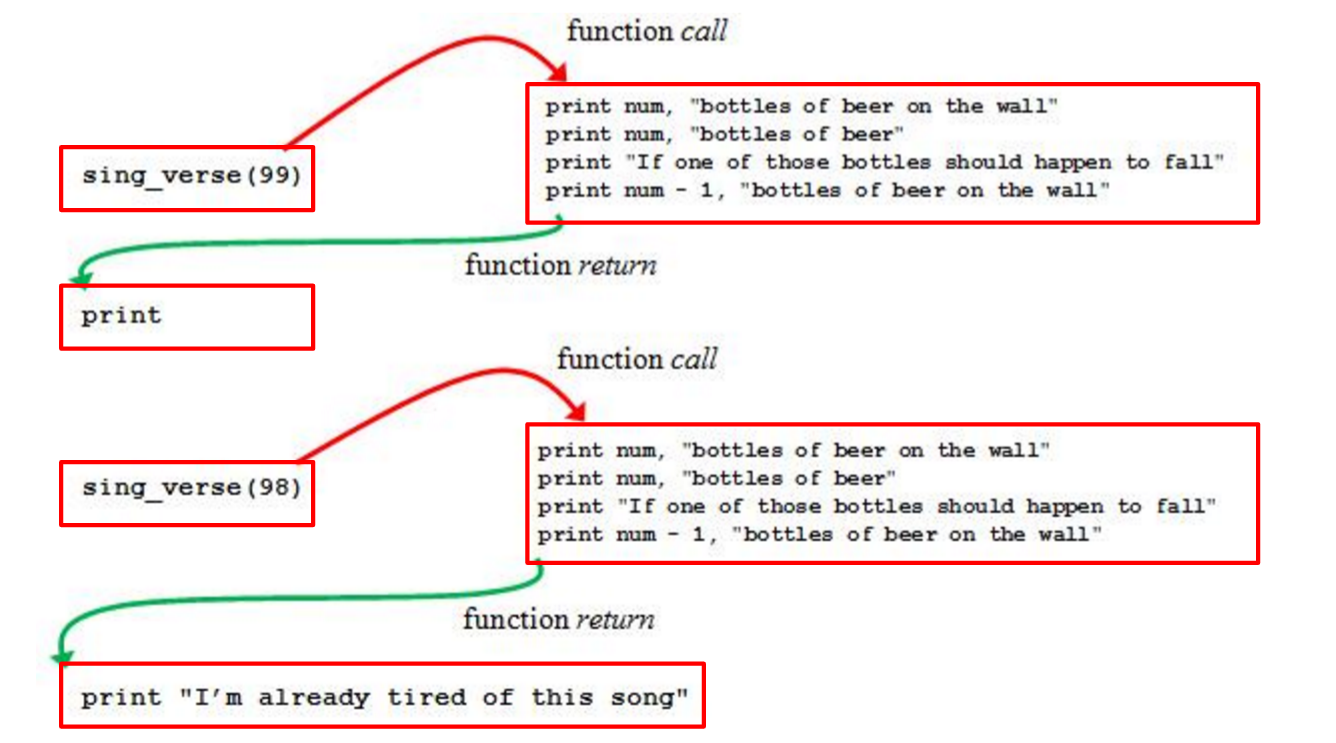We have used return statements in our value-returning functions in order to specify the value of the function. It turns out that a return statement has another purpose too: to actually cause the flow of control to literally return to the point where the function was called. For a function that doesn’t return a value, this happens automatically at the end of the function, as in sing_verse above. In the value-returning functions we have written so far, we have used a single return statement at the end of the function body, which is usually a good approach.

It is also possible to put return statements in the middle of the function body, and you should know how this works. Whenever the interpreter encounters a return statement, it stops executing the statements in the function body right then and there, and returns control to the point at which the function was called.

As an example let’s look again at the letter_grade function we wrote previously.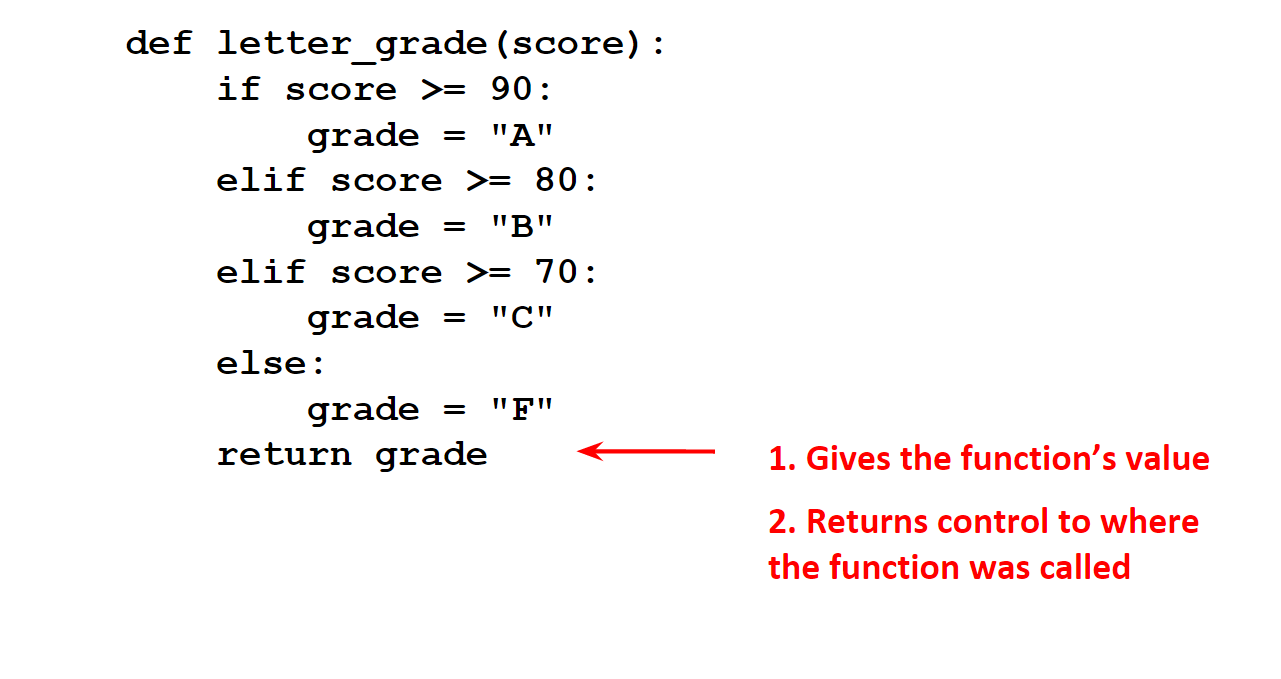Notice that there are four alternative actions (assigning a letter to the grade variable) and we’re using the elif so that exactly one of them will execute. Here is another way the function could be written.

How does this work? Let’s see what happens if the score is 85. Is 85 above 90? No, so the if-block, that is, the line return "A", is skipped. Is 85 above 80? Yes, so we execute the if-block, which contains the line return "B". The return statement does two things here: it gives the function the value “B”, and it causes the interpreter to immediately return to where the function was called. That is, the rest of the statements are not executed at all. Try this with codelens.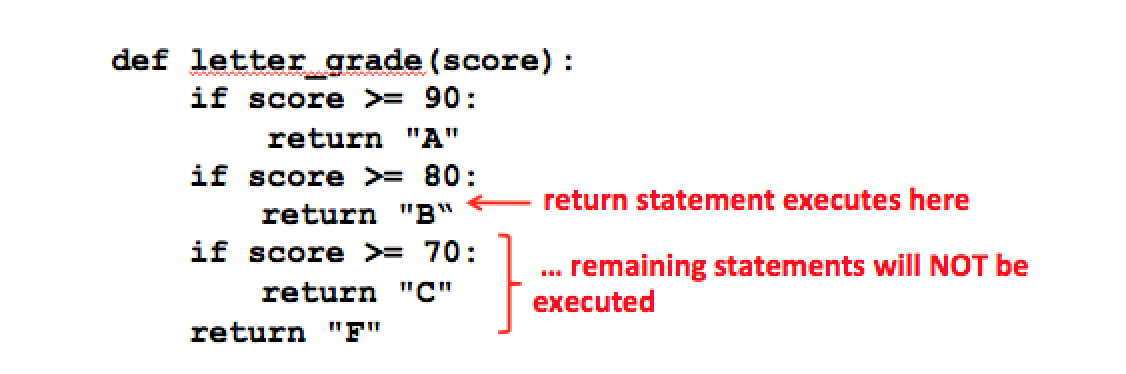For non-value-returning functions, it’s possible to use a return statement all by itself, that is, without an expression following it. This causes the interpreter to immediately return to where the function was called, but without returning a value. That is, the statement

return

is exactly the same as

return None

If a function doesn’t return a value, you normally don’t need a return statement. Returning from a function happens automatically whenever the interpreter reaches the last statement in the function. When you write a function with multiple returns, you have to be careful to make sure there’s a return statement along every possible path. For example consider this simple function for determining the maximum of two numbers, which contains a subtle error.

Try out the output. None? What happened? If first and second are the same, then neither one of the return statements will be executed. When control reaches the end of the function, it returns automatically with the value None.

Programming with multiple return statements is convenient sometimes and we will do it occasionally, especially when we start programming with loops. But for now you will probably agree that our first version of letter_grade, using elif was much easier to understand than the version above with multiple return statements. Unless there is a good reason to do otherwise, you should use a single return statement at the end of the function body.